A# orbit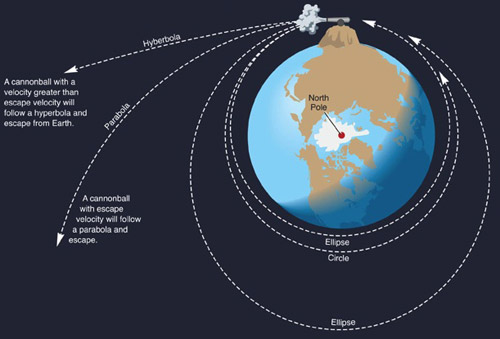Fig 1. The shape of an orbit is always one of the conic sections: circle, ellipse, parabola, or hyperbola. The orbit type depends on the velocity of the object with respect to the escape velcoity of the gravitatin body, as demonstrated by this diagram based on Newton's cannon.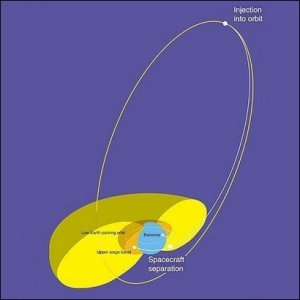Fig 2. Eccentric orbit.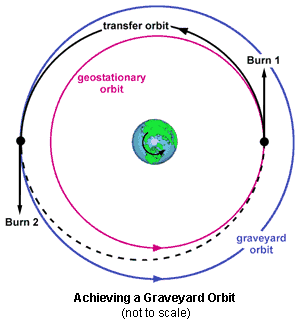Fig 3. Graveyard orbit.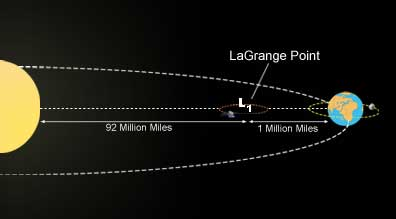Fig 4. A halo orbit around the first Lagrangian point of the Earth-Sun system.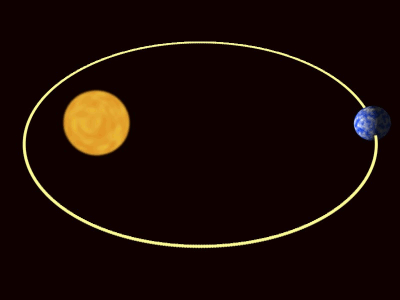Fig 5. A Keplerian orbit: Earth's path around the Sun.

An orbit is a curved path followed by an object under the gravitational influence of another body. It is one of the conic section family of curves, which includes the circle, the ellipse, the parabola and the hyperbola (Fig 1). A closed orbit, such as that followed by a satellite going around Earth, has the shape of a circle or an ellipse. An open orbit is one in which a spacecraft or other object does not follow a closed circuit around a gravitating body but simply has its path bent into the shape of a parabola or hyperbola.

As long as the orbit of an object keeps it in the vacuum of space, the object will continue to orbit without propulsive power because there is no frictional force to slow it down. If part or all of the orbit passes through Earth's atmosphere, however, the body is slowed by aerodynamic friction with the air. This causes the orbit to decay gradually to lower and lower altitudes until the object fully reenters the atmosphere and burns up.

## Orbital period

The orbital period is the time taken for a body to go once around a closed orbit. The orbital period of a planet is its "year".

For a body in an elliptical orbit of semi-major axis a about a much more massive body of mass M the orbital period P is given by

P = (2πa3/2) / √(gM).

This is the mathematical formulation of the third of Kepler's laws of planetary motion.

## Types of orbit

### circular orbit

A circular orbit is any orbit that has an eccentricity of zero – an ideal condition that is seldom if ever actually achieved. A satellite in a circular orbit travels at a constant speed. The velocity v needed to maintain such an orbit, known as the circular velocity, is given by v = √{G(M + m)/R}, where m and M are the masses of the orbiting object and the central body, respectively, R is the radius of the orbit, and G is the gravitational constant. The higher the altitude, the lower the speed, relative to the surface of Earth, needed to maintain a circular orbit.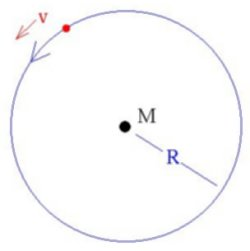### decaying orbit

A decaying orbit is one in which the orbiting object is slowly spiraling towards the primary. This is the case with Phobos, the larger martian moon, which is doomed to collide with Mars within the next few tens of millions of years.

### eccentric orbit

An eccentric orbit is a highly elliptical orbit with a low perapsis and a high apoapsis (Fig 2).

### elliptical orbit

In an elliptical orbit, the speed varies and is greatest at perigee (minimum altitude) and least at apogee (maximum altitude). Elliptical orbits can lie in any plane that passes through Earth's center.

### equatorial orbit

An equatorial orbit is one that lies in a plane passing through the equator. The angle between the orbital plane and the equator plane is called the inclination of the orbit.

### geosynchronous orbit

A geosynchronous orbit is a direct, circular, low-inclination orbit around Earth having a period of 23 h 56 min 4 sec and a corresponding altitude of 35,784 km (22,240 mi, or 6.6 Earth radii). In such an orbit, a satellite maintains a position above Earth that has the same longitude. However, if the orbit's inclination is not exactly zero, the satellite's ground-track describes a figure eight. In most cases, the orbit is chosen to have a zero inclination, and station-keeping procedures are carried out so that the spacecraft hangs motionless with respect to a point on the planet below. In this case, the orbit is said to be geostationary.

### geostationary orbit

A geostationary orbit (GSO) is a direct, circular geosynchronous orbit at an altitude of 35,786 km (22,223 mi) that lies in the plane of Earth's equator. A satellite in this orbit always appears at the same position in the sky and its ground-track is a point. Such an arrangement is ideal for some communication satellites and weather satellites since it allows one satellite to provide continuous coverage of a given area of Earth's surface.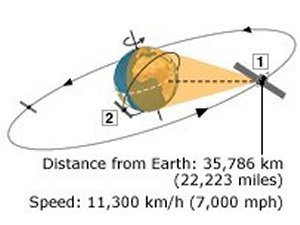1. Geostationary satellites 'parked' over equator travel at same direction and speed as Earth revolves. Each "footprint" covers 40% of globe. Directional antennae are aimed and fixed in position with no need for tracking   2. Satellites at lower orbits must travel faster than Earth revolves to avoid being pulled out of orbit by gravity, so they need tracking. Many do not follow an equatorial path

The first satellite was placed into geostationary orbit was Syncom 3 in 1964. It orbited above the Pacific Ocean and beamed pictures from the Tokyo Olympics to the US later that year – the first trans-Pacific TV transmission.

The possibility of spacecraft in geostationary orbits was first discussed by Herman Potocnik (who wrote under the pseudonym Herman Noordung) and Konstantin Tsiolkovsky. In 1945, Arthur C. Clarke discussed how a set of such satellites could form a global communications network.

### geosynchronous / geostationary transfer orbit (GTO)

A geosynchronous / geostationary transfer orbit (GTO) is an elliptical orbit, with an apogee of 35,784 km, a perigee of a few hundred km, and an inclination roughly equal to the latitude of the launch site, into which a spacecraft is initially placed before being transferred to a geosynchronous or geostationary orbit.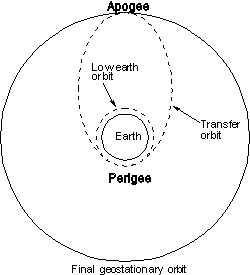After attaining GTO, the spacecraft's apogee kick motor is fired to circularize the orbit and thereby achieve the desired final orbit. Typically, this burn will also reduce the orbital inclination to 0° so that the final orbit is not only geosynchronous but also geostationary. Because the greater the initial inclination, the greater the velocity change (delta v) needed to remove this inclination, it is important that launches of GSO satellites take place as close to the equator as possible. For example, in a Delta or Atlas launch from Cape Canaveral the transfer orbit is inclined at 28.5° and the required delta v increment at apogee is 1,831 m/s; for an Ariane launch from Guiana Space Centre the inclination is 7° and the delta v is 1,502 m/s; while for a Zenit flight from the Sea Launch platform on the equator the delta v is 1,478 m/s. By the rocket equation, assuming a (typical) specific impulse of 300 sec, the fraction of the separated mass consumed by the propellant for the apogee maneuver is 46% from Cape Canaveral, 40% from Kourou, and 39% from the equator.

As a rough guide, the mass of a geostationary satellite at the start of its operational life (in GSO) is about half its initial on-orbit mass when separated from the launch vehicle (in GTO). Before carrying out the apogee maneuver, the spacecraft must be reoriented in the transfer orbit to face in the proper direction for the thrust. This reorientation is sometimes done by the launch vehicle at spacecraft separation; otherwise, it must be carried out in a separate maneuver by the spacecraft itself. In a launch from Cape Canaveral, the angle through which the satellite must be reoriented is about 132°.

### graveyard orbit

A graveyard orbit is an orbit in which a satellite, particularly a geosynchronous satellite, may be placed at the end of its operational life (see Figure 3). In a graveyard orbit, a spacecraft is not in danger of accidentally colliding with an active satellite.

### halo orbit

A halo orbit is an orbit in which a spacecraft will remain in the vicinity of a Lagrangian point, following a circular or elliptical loop around that point. The first mission to take advantage of such an orbit was ISEE-3, launched in 1978. ISEE-3 was later renamed ICE when it was sent away from L1 (the first Lagrangian point) of the Sun-Earth system to encounter a comet's tail.

Halo orbits are special cases of Lissajous orbits where the in-plane and out-of-plane frequencies are the same.

### hyperbolic orbit

A hyperbolic orbit is an open orbit in the shape of a hyperbola, the eccentricity of which is greater than 1. A hyperbolic orbit is followed by an object which escapes from the gravitational field of a larger body.

### Keplerian orbit

A Keplerian orbit is an orbit involving two spherical objects and governed by gravitational forces only (see Figure 5). Also known as a Keplerian ellipse or Keplerian trajectory, it is the path followed by an object in accordance with the first of Kepler's laws of celestial motion.

### low, medium, and high Earth orbits

low Earth orbit

Definitions of low Earth orbit (LEO) vary. According to some, LEO includes orbits having apogees and perigees between about 100 km and 1,500 km. Others extend that range up to 2,000 or 3,000 km. In some cases, the distinction between LEO and MEO (medium Earth orbit) is dropped and LEO is considered to be any orbit below geosynchronous altitude. The majority of all satellites, as well as the Space Shuttle and International Space Station, operate from LEO.

medium Earth orbit

Medium Earth orbit (MEO), also known as big LEO, is an orbit that is intermediate in altitude between that of low Earth orbit and geostationary orbit at 35,900 km.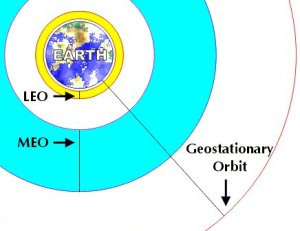high Earth orbit

HEO may stand for 'Highly elliptical orbit'. Definitions of this vary, one being an orbit with a perigee (low point) below 3,000 km and an apogee (high point) above 30,000 km.

HEO may also stand for 'High Earth orbit' – an orbit whose apogee lies above that of a geostationary orbit at 35,800 km.

### Lissajous orbit

A Lissajous orbit is a periodic orbit in which there is a combination of planar and vertical components.

### Lyapunov orbit

A Lyapunov orbit is a periodic orbit in the plane of the primary bodies. Compare with Lissajous orbit.

### parabolic orbit

A parabolic orbit is an open orbit around a central mass which is followed by an object that, at any point on its path, has the minimum velocity, known as the parabolic velocity, needed to escape from the gravitating mass (Fig 1).

### parking orbit

A parking orbit is an intermediate, waiting orbit adopted by a spacecraft between two phases of a mission. A launch vehicle boosts into the parking orbit, then coasts for a while, then fires again to enter the final desired trajectory. The alternative to a parking orbit is direct injection, where the rocket fires continuously (except during staging) until its fuel is exhausted, ending with the payload on the final trajectory .

### polar orbit

A polar orbit lies in a plane that passes through the north and south poles; in other words, it passes through Earth's axis of rotation. In other words, such an orbit has an inclination of 90 degrees. A spacecraft following such an orbit has access to virtually every point on Earth's (or some other planet's) surface, since the planet effectively rotates beneath it. This capability is especially useful for mapping or surveillance missions. An orbit at another inclination covers a smaller portion of the Earth, omitting areas around the poles. A polar orbit covers the entire globe every 14 days.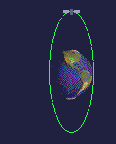A polar orbit.

Placing a satellite into terrestrial polar orbit demands more energy, and therefore more propellant, than does achieving a direct orbit of low inclination. In the latter case, the launch normally takes place near the equator, where the rotational speed of the surface contributes a significant part of the final speed needed for orbit. Since a polar orbit is not able to take advantage of the free ride provided by Earth's rotation, the launch vehicle must provide all of the energy for attaining orbital speed.

### precessing orbit

A precessing orbit, also known as a walking orbit, is one in which the orbital plane moves slowly with respect to fixed inertial space. It can be achieved intentionally by choosing the parameters of a spacecraft's orbit so that advantage is taken of some or all of the gravitational influences that give rise to precession. These factors include the deviation from perfect sphericity of a planet and the gravitational influence of other bodies such as the Sun or nearby moons.

### sun-synchronous orbit

A sun-synchronous orbit is a special case of a near-polar orbit in which a satellite, in going around Earth, passes over the same points on Earth's surface at the same local times each day and a different swathe of territory on each orbit. This kind of orbit involves passing close to both poles and crossing the meridians at a carefully-chosen angle. Sun-synchronous orbits are typically low Earth orbits with altitudes of 550 to 850 km.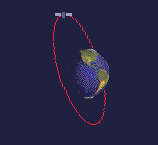A sun-synchronous orbit.

A dawn-dusk orbit is a special case of a sun-synchronous orbit in which a satellite perpetually trails the shadow of Earth cast by the Sun. Because the satellite is close to the shadow, the part of Earth's surface directly below the satellite is always at sunset or sunrise, hence the name of this type of orbit. An advantage of it is that the satellite always has its solar panels bathed in sunlight so that it can produce power by this means continuously. RADARSAT-1, for example, travels in a dawn-dusk orbit with an altitude of 798 km, period of 100.7 min, and inclination of 98.6°.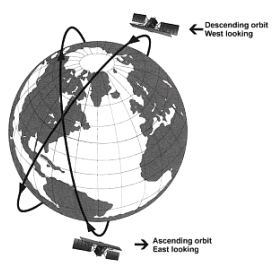Dawn-dusk orbit of RADARSAT-1.

### very high orbit

VHO (very high orbit) is a category that includes all orbits with perigees at or above geosynchronous orbit and apogees above GEO, yet remain in orbit around Earth or Earth-Moon system. Such orbits are often highly elliptical, with apogees several hundred thousand km in altitude.